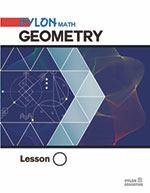# Geometry### 1. Basic Figures

Points, Lines, and Planes
Segments and Angles
Angle Pair Relationships

### 2. Basic Proof

Properties of Equality,
Midpoints, Angle Bisectors

### 3. Proofs

Properties, Postulates, Theorems
Proving Complementary Angles
Proving Supplementary Angles
Perpendicular Lines
Word Problems

### 4. Parallel Lines

Parallel Lines and Transversals
Proving Lines Parallel
Congruent Figures
SAS, ASA, SSS Postulates
Congruent Triangles

### 5. Congruent Triangles

Proving Congruent Triangles
CPCTC, Isosceles Triangles
HL Theorem
Congruent Triangles

### 6. Angles

Parallel Lines, Exterior Angle Theorem
Corresponding Parts of Triangles
Finding Perpendicular Lines
Distance Between Lines, Sum of Angles

### 7. Parallelograms

Parallelograms and Proofs
Properties of Rectangles, Rhombuses
Squares, Trapezoids
Distance Formulas

Properties of Kites,
Proportionality
Ratio and Proportion
Similar Polygons, Triangles
Midsegments

### 9. Transformations

Rigid Motion in a Plane
Reflections, Rotations
Translations and Vectors
Glide Reflections and Compositions

### 10. Right Triangles and Trigonometry

Similar Right Triangles
The Pythagorean Theorem
Special Right Triangles
Trigonometric Ratios

### 11. Circles

Tangent to Circles, Arcs and Chords
Inscribed Angles, Angle Relationships
Equations of Circles, Locus

### 12. Area of Polygons and Circles

Angle Measures in Polygons
Areas of Regular Polygons
Perimeters and Areas of Similar Figures
Circumference and Arc Length
Areas of Circles and Sectors